2014-04-29 19:57:08 u011067360 阅读数 41117
• ###### 机器学习之无监督学习三大聚类算法应用实战

本课程主要讲解介绍无监督学习 、聚类算法 、常见聚类算法的应用和优化 、tensorflow的基本处理流程。通过课程学习可以了解机器学习中的无监督学习 2.可以学习到Python下TensorFlow处理问题的流程 3.选择无监督的聚类算法来处理问题 。

2397 人正在学习 去看看 CSDN讲师

1、监督式学习（Supervised learning），是一个机器学习中的方法，可以由训练资料中学到或建立一个模式（ learning model），并依此模式推测新的实例。训练资料是由输入物件（通常是向量）和预期输出所组成。函数的输出可以是一个连续的值（称为回归分析），或是预测一个分类标签（称作分类）。

2、一个监督式学习者的任务在观察完一些训练范例（输入和预期输出）后，去预测这个函数对任何可能出现的输入的值的输出。要达到此目的，学习者必须以"合理"（见归纳偏向）的方式从现有的资料中一般化到非观察到的情况。在人类和动物感知中，则通常被称为概念学习（concept learning）。

3、监督式学习有两种形态的模型。最一般的，监督式学习产生一个全域模型，会将输入物件对应到预期输出。而另一种，则是将这种对应实作在一个区域模型。（如案例推论及最近邻居法）。为了解决一个给定的监督式学习的问题（手写辨识），必须考虑以下步骤：

1）决定训练资料的范例的形态。在做其它事前，工程师应决定要使用哪种资料为范例。譬如，可能是一个手写字符，或一整个手写的词汇，或一行手写文字。
2）搜集训练资料。这资料须要具有真实世界的特征。所以，可以由人类专家或（机器或传感器的）测量中得到输入物件和其相对应输出。
3）决定学习函数的输入特征的表示法。学习函数的准确度与输入的物件如何表示是有很大的关联度。传统上，输入的物件会被转成一个特征向量，包含了许多关于描述物件的特征。因为维数灾难的关系，特征的个数不宜太多，但也要足够大，才能准确的预测输出。
4）决定要学习的函数和其对应的学习算法所使用的数据结构。譬如，工程师可能选择人工神经网络和决策树。
5）完成设计。工程师接着在搜集到的资料上跑学习算法。可以借由将资料跑在资料的子集（称为验证集）或交叉验证（cross-validation）上来调整学习算法的参数。参数调整后，算法可以运行在不同于训练集的测试集上

1、无监督式学习(Unsupervised Learning )是人工智能网络的一种算法(algorithm)，其目的是去对原始资料进行分类，以便了解资料内部结构。有别于监督式学习网络，无监督式学习网络在学习时并不知道其分类结果是否正确，亦即没有受到监督式增强(告诉它何种学习是正确的)。其特点是仅对此种网络提供输入范例，而它会自动从这些范例中找出其潜在类别规则。当学习完毕并经测试后，也可以将之应用到新的案例上。

2、无监督学习里典型的例子就是聚类了。聚类的目的在于把相似的东西聚在一起，而我们并不关心这一类是什么。因此，一个聚类算法通常只需要知道如何计算相似度就可以开始工作了。

1、半监督学习的基本思想是利用数据分布上的模型假设, 建立学习器对未标签样本进行标签。

给定一个来自某未知分布的样本集S=L∪U, 其中L 是已标签样本集L={(x1,y1),(x2,y2), … ,(x |L|,y|L|)}, U是一个未标签样本集U={x’1,x’2,…,x’|U|},希望得到函数f:X → Y可以准确地对样本x预测其标签y，这个函数可能是参数的，如最大似然法；可能是非参数的，如最邻近法、神经网络法、支持向量机法等；也可能是非数值的，如决策树分类。其中, x与x’  均为d 维向量, yi∈Y 为样本x i 的标签, |L| 和|U| 分别为L 和U 的大小, 即所包含的样本数。半监督学习就是在样本集S 上寻找最优的学习器。如何综合利用已标签样例和未标签样例,是半监督学习需要解决的问题。

2、半监督学习问题从样本的角度而言是利用少量标注样本和大量未标注样本进行机器学习，从概率学习角度可理解为研究如何利用训练样本的输入边缘概率 P( x )和条件输出概率P ( y | x )的联系设计具有良好性能的分类器。这种联系的存在是建立在某些假设的基础上的，即聚类假设(cluster  assumption)和流形假设(maniford assumption)。

2019-11-28 19:48:59 ncepu_Chen 阅读数 89
• ###### 机器学习之无监督学习三大聚类算法应用实战

本课程主要讲解介绍无监督学习 、聚类算法 、常见聚类算法的应用和优化 、tensorflow的基本处理流程。通过课程学习可以了解机器学习中的无监督学习 2.可以学习到Python下TensorFlow处理问题的流程 3.选择无监督的聚类算法来处理问题 。

2397 人正在学习 去看看 CSDN讲师

### 有监督机器学习模型的评估指标

from sklearn.datasets import fetch_openml

# 下载minst数据集,data_home属性指定存储数据集的路径
mnist = fetch_openml('MNIST original', data_home='dataset_home')
X, y = mnist['data'], mnist['target']	# X每一行存储一张28*28的点阵图,y为对应的数字

# 展示一张图片
some_digit_image = X.reshape(28, 28)
plt.imshow(some_digit_image, cmap=matplotlib.cm.binary, interpolation='nearest')
plt.show()# 交叉验证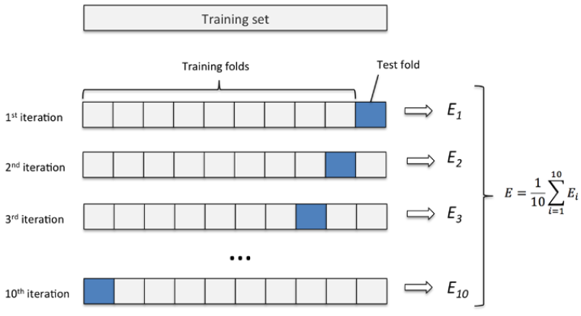• estimator: 待训练的模型.
• Xy: 训练数据.
• cv: 交叉验证的折数.
• scoring: cross_val_score()方法计算的指标.
• method: cross_val_predict()方法调用的模型的方法.
sgd_clf = SGDClassifier(loss='log')	# 创建一个逻辑回归分类器

# 进行3折交叉验证,评估其准确率
cross_val_score(sgd_clf, X_train, y_train, cv=3, scoring='accuracy')

# 进行3折交叉验证,调用分类器的predict方法进行预测
cross_val_predict(sgd_clf, X_train, y_train, cv=3, method='predict')

# 进行3折交叉验证,调用分类器的predict_proba方法得到概率值
cross_val_predict(sgd_clf, X_train, y_train, cv=3, method='predict_proba')


# 评估指标

## 混淆矩阵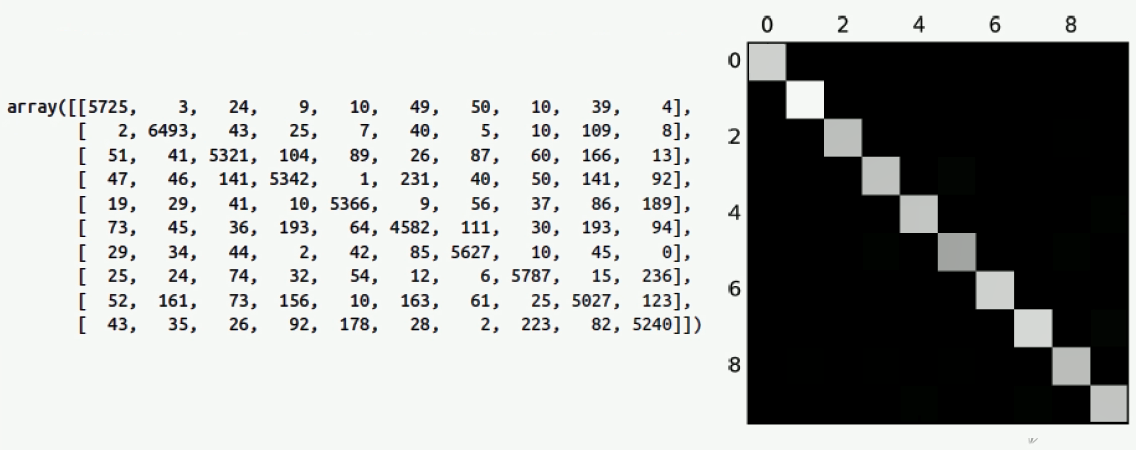print(y_true)	# array([False, False, False, ..., False, False, False])
print(y_pred)	# array([False, False, False, ..., False, False, False])

print(confusion_matrix(y_labeled, y_predict))
# 得到 array([[61413, 2274], [ 6093, 220]], dtype=int64)


## 准确率(Accuracy),精确率(Precision),召回率(Recall)

1. 准确率(Accuracy): 所有样本中被正确预测的比例.

$Accuracy = \frac{TP + TN}{TP + FP + TN + FN}$

import numpy as np
from sklearn.base import BaseEstimator
from sklearn.datasets import fetch_openml
from sklearn.linear_model import SGDClassifier
from sklearn.model_selection import cross_val_score

# 获取数据集并打乱原始数据集
mnist = fetch_openml('MNIST original', data_home='dataset_home')
X, y = mnist['data'], mnist['target']
shuffle_index = np.random.permutation(X.shape)
X_train, y_train = X[shuffle_index], y[shuffle_index]
y_train_5 = (y_train == 5)

# 创建一个逻辑回归分类器并进行交叉验证
sgd_clf = SGDClassifier(loss='log', max_iter=1000, tol=1e-4)
cross_val_score(sgd_clf, X_train, y_train_5, cv=3, scoring='accuracy')
# 进行三折交叉验证得到的准确率为 array([0.96532956, 0.96897099, 0.96018515])
cross_val_score(sgd_clf, X_train, y_train_5, cv=3, scoring='precision')
# 进行三折交叉验证得到的精确率为 array([0.95501022, 0.67672576, 0.87068966])
cross_val_score(sgd_clf, X_train, y_train_5, cv=3, scoring='recall')
# 进行三折交叉验证得到的召回率为 array([0.89643705, 0.87262357, 0.70722433])

# 创建一个无脑判负的分类器并进行交叉验证
class Never5Classifier(BaseEstimator):
def fit(self, X, y=None):
pass
def predict(self, X):
return np.zeros((len(X), 1), dtype=bool)

never_5_clf = Never5Classifier()
cross_val_score(never_5_clf, X_train, y_train_5, cv=3, scoring='accuracy')
# 进行三折交叉验证得到的准确率为 array([0.91188823, 0.90957014, 0.9079844 ])
cross_val_score(never_5_clf, X_train, y_train_5, cv=3, scoring='precision')
# 进行三折交叉验证得到的精确率为 array([0., 0., 0.])
cross_val_score(never_5_clf, X_train, y_train_5, cv=3, scoring='recall')
# 进行三折交叉验证得到的召回率为 array([0., 0., 0.])


1. 精确率(Precision): 所有预测为正例的样本中被正确预测的比例.

$Precision = \frac{TP}{TP + FP}$

2. 召回率(Recall): 所有真实值为正例的样本中被正确预测的比例.

$Recall = \frac{TP}{TP+FN}$

• 假设用户实际喜欢的所有商品(TP+FN)有10000个,推荐系统向他推荐(TP+FP)10个商品,用户最终下单(TP)了3个商品.则此时的精确率Precision为30%,召回率Recall为0.003%.
• 为了让用户多下单(增加TP),我们降低阈值(threshold),向用户推荐(TP+FP)1000个商品,用户最终下单(TP)了30个商品.则此时的精确率Precision为3%,召回率Recall为0.03%.召回率Recall上升,而精确率Precision却下降了.(这有点类似于’言多必失’的道理).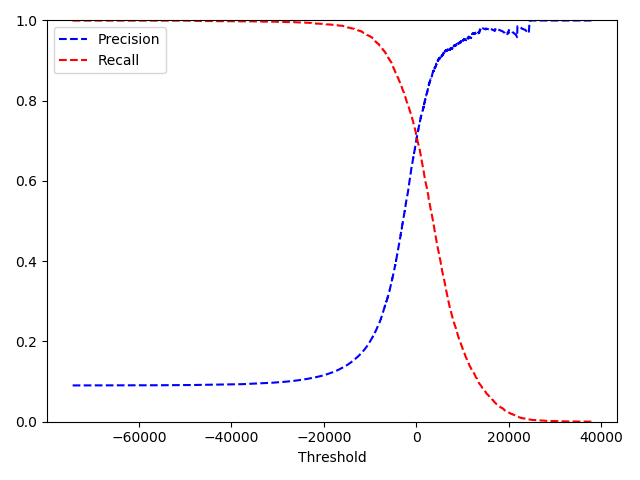# 获取逻辑回归表达式计算正例的概率
y_scores = cross_val_predict(sgd_clf, X_train, y_train, cv=3, method='decision_function')

# 生成 precisions,recalls,thresholds曲线
precisions, recalls, thresholds = precision_recall_curve(y_train, y_scores)

# 绘制 precisions,recalls,thresholds曲线
plt.plot(thresholds, precisions[:-1], 'b--', label='Precision')
plt.plot(thresholds, recalls[:-1], 'r--', label='Recall')
plt.xlabel("Threshold")
plt.legend(loc='upper left')
plt.ylim([0, 1])
plt.show()


## F1-score

F1-Score被定义为精确率Precision召回率Recall调和平均数:

$F_1 = \frac{2}{\frac{1}{Precision}+\frac{1}{Recall}} = 2 \cdot \frac{Precision \times Recall}{Precision + Recall}$

$F_{\beta} = \frac{1+\beta^2}{\frac{1}{Precision} + \frac{\beta^2}{Recall}} = (1+\beta^2) \cdot \frac{Precision \times Recall}{\beta^2 \, Precision+Recall}$

## AUC

ROC(receiver operating characteristic)曲线.ROC曲线上每个点反映着对同一信号刺激的感受性,即在不同阈值(threshold)下的负正类率(False Positive Rate,FPR)和真正类率(True Positive Rate,TPR).

• 真正类率TPR表示所有真实值为正例的样本中,被正确预测为正例的样本的比例.

$TPR = \frac{TP}{TP+FN}$

• 负正类率FPR表示所有真实值为负例的样本中,被错误预测为正例的样本的比例.

$FPR = \frac{FP}{FP+TN}$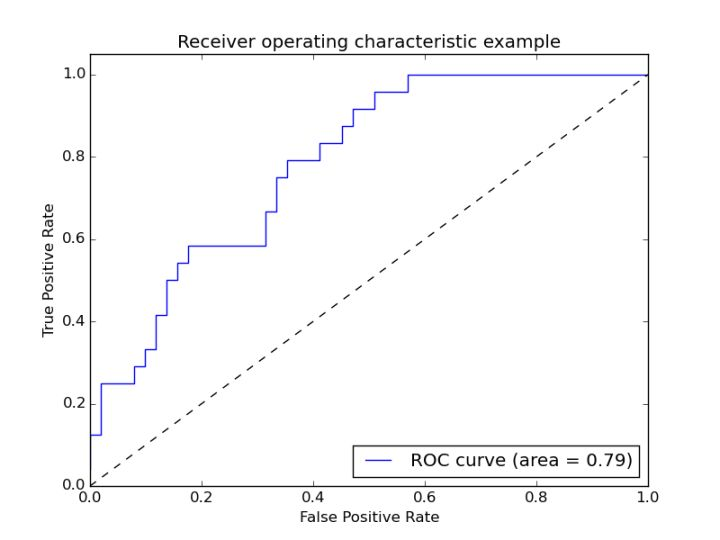AUC(Area under Curve)为ROC曲线下方的面积,即TPR对FPR积分的结果.容易看出AUC更看重正例.

# 使用逻辑回归分类器计算正例的概率
sgd_clf = SGDClassifier(loss='log', max_iter=1000, tol=1e-4)
y_scores_sgd = cross_val_predict(sgd_clf, X_train, y_train, cv=3, method='decision_function')
fpr_sgd, tpr_sgd, thresholds_sgd = roc_curve(y_train, y_scores)

# 使用随机森林分类器计算正例的概率
forest_clf = RandomForestClassifier(random_state=42)
y_probas_forest = cross_val_predict(forest_clf, X_train, y_train, cv=3, method='predict_proba')
y_scores_forest = y_probas_forest[:, 1]
fpr_forest, tpr_forest, thresholds_forest = roc_curve(y_train, y_scores_forest)

# 绘制ROC曲线
plt.plot(fpr_sgd, tpr_sgd, 'b:', label='SGD(AUC=%.5f)' % roc_auc_score(y_train, y_scores_sgd))
plt.plot(fpr_forest, tpr_forest, label='Random Forest(AUC=%.5f)' % roc_auc_score(y_train, y_scores_forest))
plt.legend(loc='lower right')
plt.show()2019-08-03 21:17:26 qq_41386300 阅读数 41
• ###### 机器学习之无监督学习三大聚类算法应用实战

本课程主要讲解介绍无监督学习 、聚类算法 、常见聚类算法的应用和优化 、tensorflow的基本处理流程。通过课程学习可以了解机器学习中的无监督学习 2.可以学习到Python下TensorFlow处理问题的流程 3.选择无监督的聚类算法来处理问题 。

2397 人正在学习 去看看 CSDN讲师

### 有监督机器学习训练流程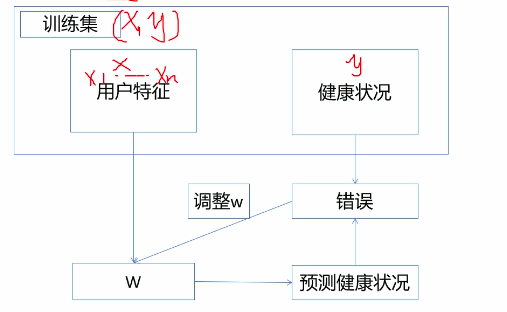• 我们的目标就是得到模型，也就是参数，
例如 y=a+b*x， a和b就是参数，我们一般用W（w0…wn)表示，w有两部分，w0以及w1…wn，
w1…wn对应x1…xn。

x1+w2x2+…+wnxn

• 训练过程
刚开始不知道w是什么的时候，先初始化，也就是随机生成一些值，
把x和w代入计算会得到一些值，我们叫y^
有了y^,我们就可以评估当前的w好还是不好，误差=| y ^-y|
我们根据误差不断的调整w使得误差越来越小（调整方法有很多，以后再学）
把调整后的w再带入计算得到新的y^,然后得到新的误差，再次调整w，依次类推…直到误差不变的时候，说明此时的w是最优的

2018-12-30 21:50:51 daerzei 阅读数 2775
• ###### 机器学习之无监督学习三大聚类算法应用实战

本课程主要讲解介绍无监督学习 、聚类算法 、常见聚类算法的应用和优化 、tensorflow的基本处理流程。通过课程学习可以了解机器学习中的无监督学习 2.可以学习到Python下TensorFlow处理问题的流程 3.选择无监督的聚类算法来处理问题 。

2397 人正在学习 去看看 CSDN讲师

## 前言

机器学习是数据分析和数据挖掘的一种比较常用，比较好的手段从有无监督的角度，可以分为三类：

1. 有监督学习
2. 无监督学习
3. 半监督学习

## 有监督学习

用已知某种或某些特性的样本作为训练集，以建立一个数学模型，再用已建立的模型来预测未知样本，此种方法被称为有监督学习，是最常用的一种机器学习方法。是从标签化训练数据集中推断出模型的机器学习任务

问：有监督学习的定义最关键的是什么呢?
从标签化的训练数据集中推断出模型机器学习任务
前面都是废话，没有用，最关键的要看的就是:从标签化的训练数据集中推断出模型机器学习任务

问：什么是标签
比如说有一张猫的图片，我们都知道它是只猫，但是计算机不知道它是只猫，怎么办呢?给这张图片打上个标签，然后计算机就知道它是只猫了，后面计算机根据这个标签对所有的猫进行学习就能通过这一只猫认识所有的猫了。

给数据打标签，其实就是告诉计算机，前面这么多特征（$x_1$ ~ $x_n$)到底描述的是什么。很自然的就是Y，Y可以称之为Label，也可以称之为Target(我们的目标值)

我们接到一个任务或者说模型，首先要定义一个目标，这个目标对应的是谁?我们的目标要对这个任务进行合理的建模和预测，也就是说我们的目标在进行预测的时候有一个对应的y的值的话，
x1第一个特征，xn第n个特征，矩阵一般是有m行n列的这样一个矩阵，所以我们一般去写的时候，这个数据集中一般会有m条观测值以及n列的这样一个特征。

然后最终会有一个目标值的y，如果有y，我们就称之为有监督学习，我们就要使用有监督学习进行模型的构建，实际上我们在日常的业务当中能够多使用有监督学习就多使用有监督学习，比如说在有$x_1, x_2, x_3, \cdots, x_n$以及y的情况下就老老实实用有监督学习了，就别用无监督学习。

## 无监督学习

知道了有监督学习的定义了，无监督学习的定义也就出来了。在算法构建的过程中不考虑Y的值，只通过特征信息去归纳出一些新的规律出来，这个方法就称之为无监督学习。

有监督学习和无监督学习的区别就是一个有y，一个没有y。这是最简单的记忆方式。

所以总结起来正式的说法是:无监督学习的训练集中没有人为的标注的结果，在非监督的学习过程中，数据并不被特别标识，学习模型是为了推断出数据的一些内存结构。

推断出数据的一些内在结构这是无监督学习学习的作用

问：为什么不建议使用无监督学习呢
无监督学习需要我们推断一些结构，但是在推断一些结构的时候可能会因为人为的主观臆断而出现一些偏差，这个就不是纯数学能够证明我是对的了，这个时候再进行无监督学习就是在瞎猜。

在没有y值的情况怎么办?是不是只能进行无监督学习了呢?
没有Y值的时候，自己给数据打标签，自己把Y做出来。然后进行有监督学习
很多情况下，在实际的工作业务中很多的工作量都是给数据打标签，你可能花半个半个月的时间，五六个人就坐那儿天天打标签，几万条数据坐那儿打标签打个三四天很正常。

所以就需要注意了，如果说真的是说在没有标签的情况下，你看看有没有合理的方式先把标签获取过来。 如果没有合理的渠道，就看看能不能进行人为地打标签再进行有监督学习的学习，这样做的结果会更好些。

## 半监督学习

看上面有监督学习和无监督学习的定义，就是一半有一半无呗
意思就是用少量的有标注的样本和大量未标注的样本进行训练和分类，这样是有监督学习和无监督学习的结合。

考虑如何利用少量的瓢样本和大量的未瓢样本进行训练和分类的问题，
是有监督学习和无监督学习的结合。

这就是机器学习大致的一个分类

有监督 机器学习 相关内容

阅读数 5302

博文 来自： starzhou

阅读数 428

博文 来自： yeziand01

阅读数 1209

博文 来自： qq_42730789

阅读数 1980

博文 来自： hajk2017
没有更多推荐了，返回首页